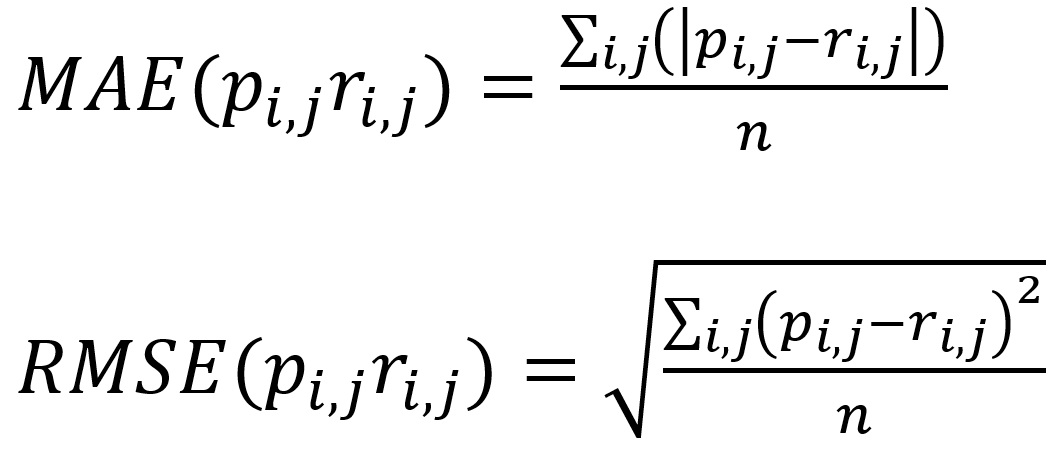# Evaluation Metrics

Two main categories are used to decide on the quality of collaborative filtering algorithms:

• Predictive accuracy metrics (for example Mean Absolute Error and its variations, F1-measure, and recall);
• Receiver Operation Characteristic (ROC) sensitivity (for example, Pearson’s product-moment correlation, Mean Average Precision, half-life utility, Kendalls’ Tau, and normalized distance-based performance metric).

The most widely used evaluation metrics in CF are the Mean Absolute Error (MAE), and the Root Mean Squared Error (RMSE). The equations are as follows:Where n is the total number of ratings, pi,j is the predicted rating for user i on item j, and rij is the actual rating.